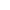# 题解 | #密码强度等级#

https://www.nowcoder.com/practice/52d382c2a7164767bca2064c1c9d5361

```# range(x, y) includes x up to but not including y.
# So range(5, 7) is 5, 6 but not 7.

# !"#\$%&'()*+,-./:;<=>?@[\]^_`{|}~
# !"#\$%&\'()*+,-./:;<=>?@[\\]^\_`{|}~
# '\_ needs to be escaped

def length(s):
if len(s) <= 4:
return 5
elif len(s) in range(5, 8):
return 10
else:
return 25

def alpha(s):
has_upper = 0
has_lower = 0
for char in s:
if char.isupper():
has_upper = 10
elif char.islower():
has_lower = 10
return has_upper + has_lower

def digit(s):
s2 = 0
c2 = 0
for char in s:
if char.isdigit():
s2 = 10
c2 += 1
if c2 > 1:
s2 = 20
break
return s2

def symbol(s):
symbols = '!"#\$%&\'()*+,-./:;<=>?@[\\]^\_`{|}~'
s3 = 0
c3 = 0
for char in s:
if char in symbols:
s3 = 10
c3 +=1
if c3 > 1:
s3 = 25
break
return s3

def bonus(s):
s4 = 0
if alpha(s) and digit(s):
s4 = 2
if symbol(s):
s4 = 3
if alpha(s) == 20:
s4 = 5
return s4

def level(s):
if s in range(0, 24):
print('VERY_WEAK')
elif s in range(25, 50):
print('WEAK')
elif s in range(50, 60):
print('AVERAGE')
elif s in range(60, 70):
print('STRONG')
elif s in range(70, 80):
print('VERY_STRONG')
elif s in range(80, 90):
print('SECURE')
else:
print('VERY_SECURE')

pw = input()
score = length(pw) + alpha(pw) + digit(pw) + symbol(pw) + bonus(pw)
level(score)

# print(str(score), str(length(pw)), str(alpha(pw)), str(digit(pw)), str(symbol(pw)), str(bonus(pw)))
```
• 符号判断遇到了些阻碍，Claude用的是string.punctuation
• 创建一个字符串列表后还需要用\来Escape可能冲突的符号
• 对range()的边界条件不熟悉，它是不包括上边界的